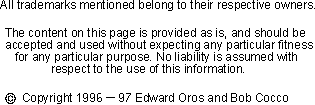Design Your Own 5/8 Wave Vertical Antenna

The 5/8 wave antenna consists of a vertical radiator which is fed at the base of the antenna. A matching device of some sort must be added between the antenna and the feedline if you wish to feed it with coax. Adding a coil in series with the antenna at the base is one such method of matching.

So why would anyone use a 5/8 wave antenna if they have to go through all that extra work? After all, a ground plane antenna provides a nicer match. There are a couple of answers. The first is GAIN. The computer shows that the antenna (mounted 1 foot above ground) has a gain of about 1.5 dBd higher than a dipole's gain (also mounted 1 foot above ground.)

The second reason you may want to use the 5/8 wave vertical is to obtain a lower angle of radiation. A half wave antenna's radiation peak angle is 20 degrees. You'll find that the 5/8 wave antenna's angle of radiation is just 16 degrees making it an even better dx antenna.

You may have noticed a pattern developing here. A quarter wave ground plane antenna has a radiation pattern that produces maximum gain at about 25 degrees and a half wave antenna drops that angle to 20 degrees, and the 5/8 wave antenna further drops that angle to 16 degrees. So why not just keep extending the antenna out to one full wave? Well it would be nice if it worked but unfortunately the wave pattern begins to create very high angles of radiation beyond 5/8 wave. So we've reached the maximum gain at this point and extending the antenna any further just reduces the gain where we want it (low angles). Of course if you are interested in very short skip, extending the antenna will produce nice gains over a dipole.

All antenna lengths depend on various factors. Some of these factors are:the height above ground, the diameter of the wire, nearby structures, the effects of other antennas in the area and even the conductivity of the soil.

This page allows you to calculate the length for a 5/8 wave antenna. It uses the standard formula, 585/f (178.308/f for metric) MHz to calculate the element lengths. If you've experimented with 5/8 wave antennas before and know of a better formula for your QTH, feel free to change the formula to suit. This formula is for a wire antenna. Of course if you construct your antenna out of tubing, the total length of the antenna will be shorter, for example I've found that 21.5 feet seems to give the maximum gain for a frequency of 28.5 MHz when using 1" tubing. And 22.5 feet seems to be the best length for wire at the same frequency. Since the formula calculates the antenna to be about 2 foot shorter, be sure to experiment and perhaps add a little to your final length.
Enter the formula for the antenna calculation

Switch to:

Divided by Freq MHz

Your 5/8 wave antenna's vertical length is

Back to the Antenna Elmer